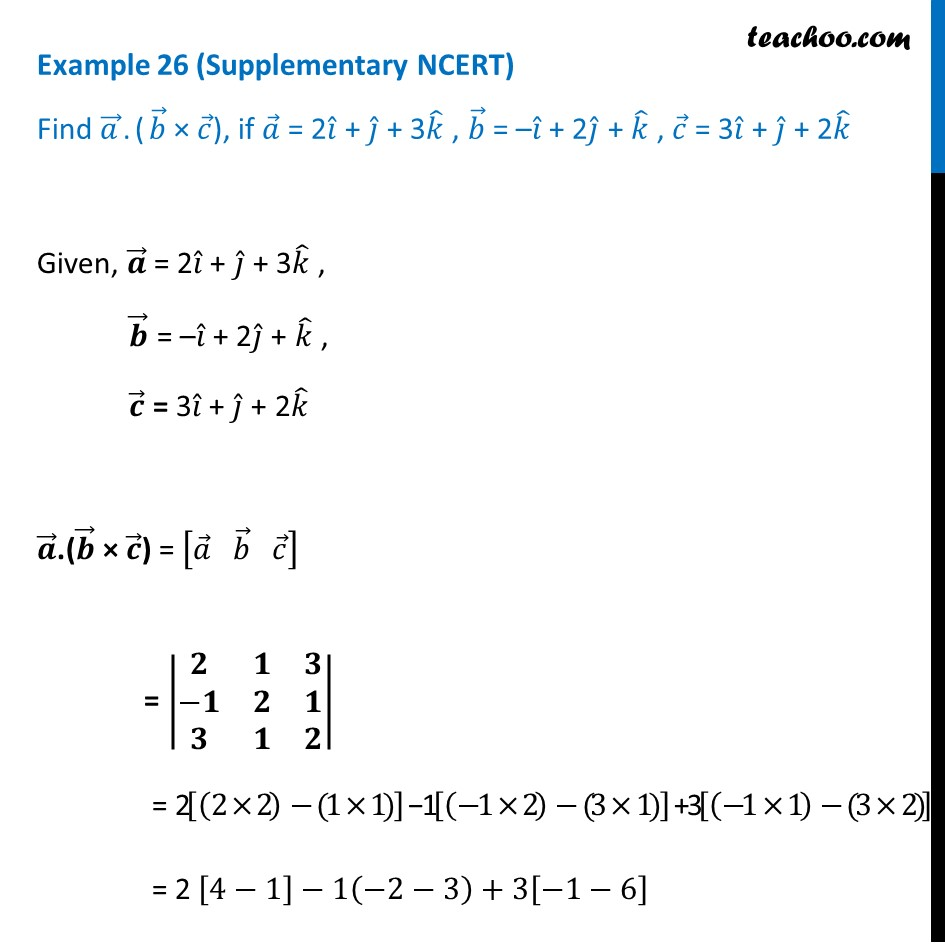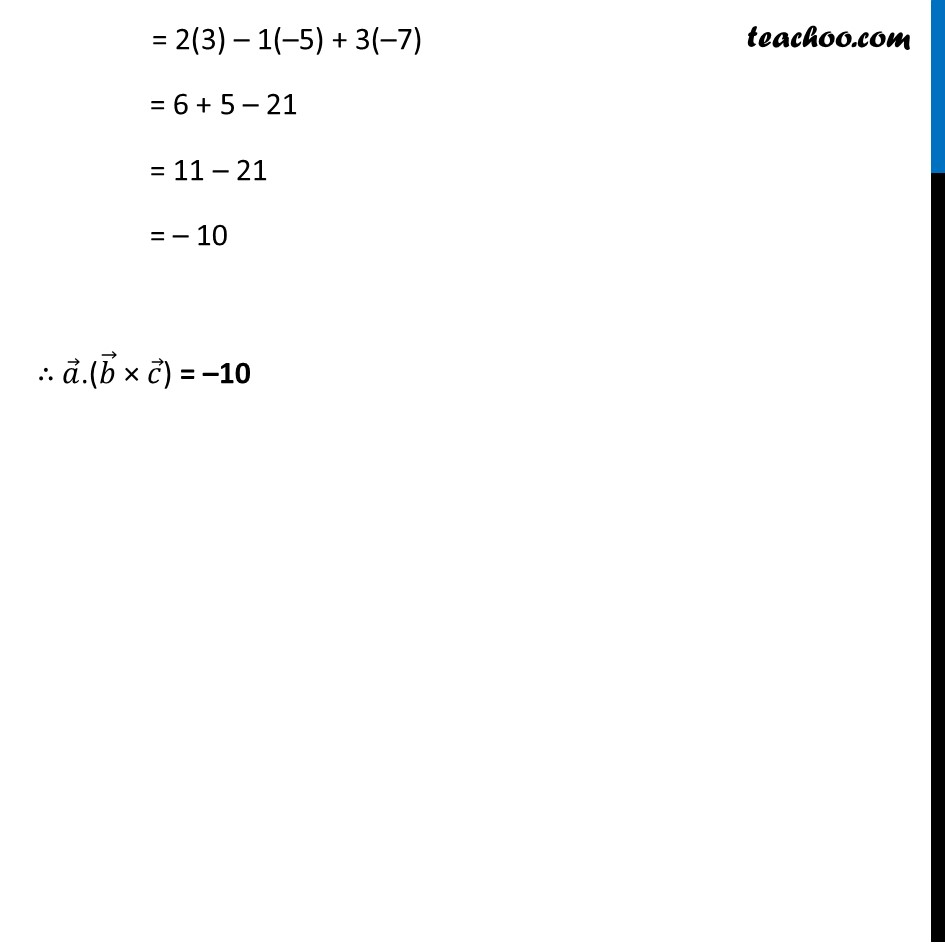1. Chapter 10 Class 12 Vector Algebra (Term 2)
2. Serial order wise
3. Examples

Transcript

Example 26 (Supplementary NCERT) Find 𝑎 ⃗.(𝑏 ⃗ × 𝑐 ⃗), if 𝑎 ⃗ = 2𝑖 ̂ + 𝑗 ̂ + 3𝑘 ̂ , 𝑏 ⃗ = –𝑖 ̂ + 2𝑗 ̂ + 𝑘 ̂ , 𝑐 ⃗ = 3𝑖 ̂ + 𝑗 ̂ + 2𝑘 ̂Given, 𝒂 ⃗ = 2𝑖 ̂ + 𝑗 ̂ + 3𝑘 ̂ , 𝒃 ⃗ = –𝑖 ̂ + 2𝑗 ̂ + 𝑘 ̂ , 𝒄 ⃗ = 3𝑖 ̂ + 𝑗 ̂ + 2𝑘 ̂ 𝒂 ⃗.(𝒃 ⃗ × 𝒄 ⃗) = [𝑎 ⃗" " 𝑏 ⃗" " 𝑐 ⃗ ] = |■8(𝟐&𝟏&𝟑@−𝟏&𝟐&𝟏@𝟑&𝟏&𝟐)| = 2[(2×2)−(1×1) ] − 1[(−1×2)−(3×1) ] + 3[(−1×1)−(3×2)] = 2 [4−1]−1(−2−3)+3[−1−6] = 2(3) – 1(–5) + 3(–7) = 6 + 5 – 21 = 11 – 21 = – 10 ∴ 𝑎 ⃗.(𝑏 ⃗ × 𝑐 ⃗) = –10

Examples

Chapter 10 Class 12 Vector Algebra (Term 2)
Serial order wise